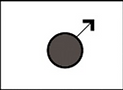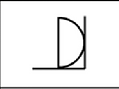Đề 2Question 1. Which set does the Figure belong to?Question 2. Which comes next in the sequence?Question 3. What replaces the question mark?Question 4. What replaces the question mark?Question 5. Which set dose the Figure belong to?Question 6. Which comes next in the sequence?Question 7. What replaces the question mark?Question 8. What replaces the question mark?Question 9. Which set dose the Figure belong to?Question 10. Which comes next in the sequence?Question 11. Which set dose the Figure belong to?Question 12. Which comes next in the sequence?Question 13. What replaces the question mark?Question 14. Which set dose the Figure belong to?Question 15. Which comes next in the sequence?II. NUMBERICQuestion 2. What were the total production costs for 2009 (to the nearest £100,000)?Question 4. What percent of total costs did Property depreciation represent in 2007?Question 7. Brand A and Brand D are to have their number of staff reduced by the same percentage reduction seen by their Marketing Budgets between 2010 and 2011. If the number of staff at Brand A was originally 120 and the number of staff at Brand D triple this, what are the new reduced staff numbers for each Brand?III. VERBALQuestion 3. Bitumen is a highly viscous form of petroleum that needs to be heated to flow.Question 6. A 2009 study linking CFS with a retrovirus has now been discredited.